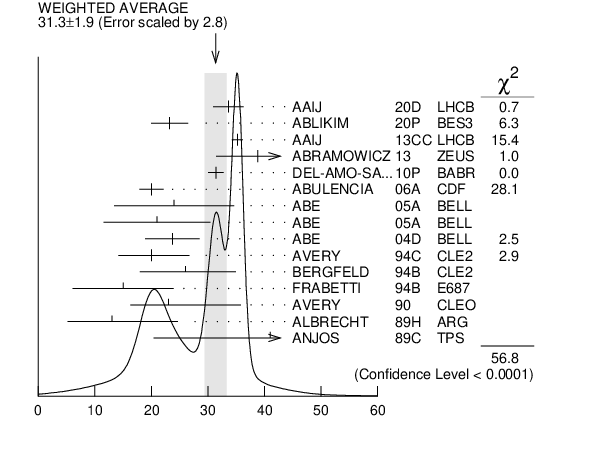${{\boldsymbol D}_{{1}}{(2420)}}$ WIDTH

VALUE (MeV) EVTS DOCUMENT ID TECN CHG  COMMENT
 $\bf{ 31.3 \pm1.9}$ OUR AVERAGE  Error includes scale factor of 2.8. See the ideogram below.
$33.6$ $\pm0.3$ $\pm2.7$ 79k 1
 2020 D
LHCB 0 ${{\mathit B}^{-}}$ $\rightarrow$ ${{\mathit D}^{*+}}{{\mathit \pi}^{-}}{{\mathit \pi}^{-}}$
$23.2$ $\pm2.3$ $\pm2.3$ 4207
 2020 P
BES3 + ${{\mathit e}^{+}}$ ${{\mathit e}^{-}}$ $\rightarrow$ ${{\mathit D}^{+}}{{\mathit D}^{-}}{{\mathit \pi}^{+}}{{\mathit \pi}^{-}}$
$35.2$ $\pm0.4$ $\pm0.9$ 210k
 2013 CC
LHCB 0 ${{\mathit p}}$ ${{\mathit p}}$ $\rightarrow$ ${{\mathit D}^{*+}}{{\mathit \pi}^{-}}{{\mathit X}}$
$38.8$ $\pm5.0$ ${}^{+1.9}_{-5.4}$ 2.7k 2
 2013
ZEUS 0 ${{\mathit e}^{\pm}}$ ${{\mathit p}}$ $\rightarrow$ ${{\mathit D}^{(*)+}}{{\mathit \pi}^{-}}{{\mathit X}}$
$31.4$ $\pm0.5$ $\pm1.3$ 103k
 2010 P
BABR 0 ${{\mathit e}^{+}}$ ${{\mathit e}^{-}}$ $\rightarrow$ ${{\mathit D}^{*+}}{{\mathit \pi}^{-}}{{\mathit X}}$
$20.0$ $\pm1.7$ $\pm1.3$ 7.5k
 2006 A
CDF 0 1900 ${{\mathit p}}$ ${{\overline{\mathit p}}}$ $\rightarrow$ ${{\mathit D}^{*+}}{{\mathit \pi}^{-}}{{\mathit X}}$
$24$ $\pm7$ $\pm8$ 151
 2005 A
BELL 0 ${{\mathit B}^{-}}$ $\rightarrow$ ${{\mathit D}^{0}}{{\mathit \pi}^{+}}{{\mathit \pi}^{-}}{{\mathit \pi}^{-}}$
$21$ $\pm5$ $\pm8$ 124
 2005 A
BELL + ${{\overline{\mathit B}}^{0}}$ $\rightarrow$ ${{\mathit D}^{+}}{{\mathit \pi}^{+}}{{\mathit \pi}^{-}}{{\mathit \pi}^{-}}$
$23.7$ $\pm2.7$ $\pm4.0$ 3
 2004 D
BELL 0 ${{\mathit B}^{-}}$ $\rightarrow$ ${{\mathit D}^{*+}}{{\mathit \pi}^{-}}{{\mathit \pi}^{-}}$
$20$ ${}^{+6}_{-5}$ $\pm3$ 286
 1994 C
CLE2 0 ${{\mathit e}^{+}}$ ${{\mathit e}^{-}}$ $\rightarrow$ ${{\mathit D}^{*+}}{{\mathit \pi}^{-}}$ X
$26$ ${}^{+8}_{-7}$ $\pm4$ 146
 1994 B
CLE2 + ${{\mathit e}^{+}}$ ${{\mathit e}^{-}}$ $\rightarrow$ ${{\mathit D}^{*0}}{{\mathit \pi}^{+}}$ X
$15$ $\pm8$ $\pm4$ 51
 1994 B
E687 0 ${{\mathit \gamma}}$ ${}^{}\mathrm {Be}$ $\rightarrow$ ${{\mathit D}^{*+}}{{\mathit \pi}^{-}}$ X
$23$ ${}^{+8}_{-6}$ ${}^{+10}_{-3}$ 279
 1990
CLEO 0 ${{\mathit e}^{+}}$ ${{\mathit e}^{-}}$ $\rightarrow$ ${{\mathit D}^{*+}}{{\mathit \pi}^{-}}$ X
$13$ $\pm6$ ${}^{+10}_{-5}$ 171
 1989 H
ARG 0 ${{\mathit e}^{+}}$ ${{\mathit e}^{-}}$ $\rightarrow$ ${{\mathit D}^{*+}}{{\mathit \pi}^{-}}$ X
$41$ $\pm19$ $\pm8$ 190
 1989 C
TPS + ${{\mathit \gamma}}$ ${{\mathit N}}$ $\rightarrow$ ${{\mathit D}^{0}}{{\mathit \pi}^{+}}{{\mathit X}^{0}}$
• • We do not use the following data for averages, fits, limits, etc. • •
$53.2$ $\pm7.2$ ${}^{+3.3}_{-4.9}$ 3.1k
 2009
ZEUS 0 ${{\mathit e}^{\pm}}$ ${{\mathit p}}$ $\rightarrow$ ${{\mathit D}^{*+}}{{\mathit \pi}^{-}}{{\mathit X}}$
$58$ $\pm14$ $\pm10$ 171
 1989 C
TPS 0 ${{\mathit \gamma}}$ ${{\mathit N}}$ $\rightarrow$ ${{\mathit D}^{*+}}{{\mathit \pi}^{-}}$ X
 1 From a full four-body amplitude analysis of the ${{\mathit B}^{-}}$ $\rightarrow$ ${{\mathit D}^{*+}}{{\mathit \pi}^{-}}{{\mathit \pi}^{-}}$ decay.
 2 From the combined fit of the ${{\mathit M}}$( ${{\mathit D}^{+}}{{\mathit \pi}^{-}}$ ) and ${{\mathit M}}$( ${{\mathit D}^{*+}}{{\mathit \pi}^{-}}$ ) distributions. and A$_{{{\mathit D}_{{2}}}}$ fixed to the theoretical prediction of $-1$.
 3 Fit includes the contribution from ${{\mathit D}_{{1}}^{*}{(2430)}^{0}}$.${{\mathit D}_{{1}}{(2420)}}$ WIDTH (MeV)
References:
 AAIJ 2020D
PR D101 032005 Determination of quantum numbers for several excited charmed mesons observed in $B^- \to D^{*+} \pi^- \pi^-$ decays
 ABLIKIM 2020P
PL B804 135395 Study of $e^{+}e^{-} \to D^{+} D^{-} \pi^{+} \pi^{-}$ at center-of-mass energies from 4.36 to 4.60 GeV
 AAIJ 2013CC
JHEP 1309 145 Study of ${{\mathit D}_{{J}}}$ Meson Decays to ${{\mathit D}^{+}}{{\mathit \pi}^{-}}$, ${{\mathit D}^{0}}{{\mathit \pi}^{+}}$ and ${{\mathit D}^{*+}}{{\mathit \pi}^{-}}$ Final States in ${{\mathit p}}{{\mathit p}}$ Collisions
 ABRAMOWICZ 2013
NP B866 229 Production of the Excited Charm Mesons ${{\mathit D}_{{1}}}$ and ${{\mathit D}_{{2}}^{*}}$ at HERA
 DEL-AMO-SANCHEZ 2010P
PR D82 111101 Observation of New Resonances Decaying to ${{\mathit D}}{{\mathit \pi}}$ and ${{\mathit D}^{*}}{{\mathit \pi}}$ in Inclusive ${{\mathit e}^{+}}{{\mathit e}^{-}}$ Collisions near $\sqrt {s }$ = 10.58 GeV
 CHEKANOV 2009
EPJ C60 25 Production of Excited Charm and Charm-Strange Mesons at HERA
 ABULENCIA 2006A
PR D73 051104 Measurement of Mass and Width of the Excited Charmed Meson States ${{\mathit D}_{{1}}^{0}}$ and ${{\mathit D}_{{2}}^{*0}}$ at CDF
 ABE 2005A
PRL 94 221805 Observation of the ${{\mathit D}_{{1}}{(2420)}}$ $\rightarrow$ ${{\mathit D}}{{\mathit \pi}^{+}}{{\mathit \pi}^{-}}$ Decays
 ABE 2004D
PR D69 112002 Study of ${{\mathit B}^{-}}$ $\rightarrow$ ${{\mathit D}^{**0}}{{\mathit \pi}^{-}}$ ( ${{\mathit D}^{**0}}$ $\rightarrow$ ${{\mathit D}}{}^{(*)+}$ ${{\mathit \pi}^{-}}$ ) Decays
 AVERY 1994C
PL B331 236 Production and Decay of ${{\mathit D}_{{1}}{(2420)}^{0}}$ and ${{\mathit D}_{{2}}^{*}{(2460)}^{0}}$
 BERGFELD 1994B
PL B340 194 Observation of ${{\mathit D}_{{1}}{(2420)}^{+}}$ and ${{\mathit D}_{{2}}^{*}{(2460)}^{+}}$
 FRABETTI 1994B
PRL 72 324 Measurement of the Masses and Widths of $\mathit L$ = 1 Charm Mesons
 AVERY 1990
PR D41 774 P-wave Charmed Mesons in ${{\mathit e}^{+}}{{\mathit e}^{-}}$ Annihilation
 ALBRECHT 1989H
PL B232 398 Resonance Decomposition of the ${{\mathit D}^{*}{(2420)}^{0}}$ Through a Decay Angular Analysis
 ANJOS 1989C
PRL 62 1717 Observation of Excited Charmed Mesons